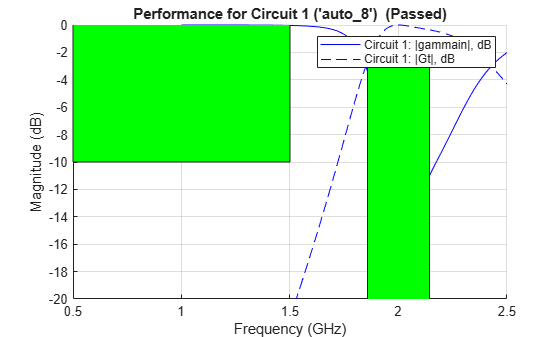Adds performance goal for sort, pass, or fail matching network design

## Syntax

``mnobjupdated = addEvaluationParameter(mnobj,parameter,comparison,targetdb,band,weight)``

## Description

example

````mnobjupdated = addEvaluationParameter(mnobj,parameter,comparison,targetdb,band,weight)` adds a performance goal to an existing matching network and returns an updated matching network object.```

## Examples

collapse all

Create a dipole antenna and create the S-parameters of the antenna. This example requires Antenna Toolbox.

```d = dipole('Length', 0.103, 'Width',0.0022); freq = linspace(0.5e9,2.5e9,1001); sd = sparameters(d, freq);```

Alternatively, load S-Parameters from the MAT file

`% load('sparams_dipole.mat')`

Create a matching network from the S-parameters.

```n = matchingnetwork('LoadImpedance',sd,'Components',3,... 'LoadedQ',7,'CenterFrequency',2e9);```

Get the evaluation parameters of the network.

`t = getEvaluationParameters(n)`
```t=1×6 table Parameter Comparison Goal Band Weight Source _________ __________ ______ _________________________ ______ _____________ {'Gt'} {'>'} {[-3]} {[1.8571e+09 2.1429e+09]} {} {'Automatic'} ```

Plot the reflection coefficient and transducer gain of the matching network circuit 1 , at a frequency range of 1 GHz to 2.5 GHz.

`rfplot(n, (1e9:0.001e9:2.5e9),1);`Add a new evaluation parameter to compare the transducer gain to have a cut-off of less than -10 dB. Use a frequency range of 0.5 GHz to 1.5 GHz. Plot the comparisons.

```n = addEvaluationParameter(n, 'Gt', '<', -10, [0.5e9 1.5e9], 1); t = getEvaluationParameters(n)```
```t=2×6 table Parameter Comparison Goal Band Weight Source _________ __________ _______ _________________________ ______ __________________ {'Gt'} {'>'} {[ -3]} {[1.8571e+09 2.1429e+09]} {} {'Automatic' } {'Gt'} {'<'} {[-10]} {[ 500000000 1.5000e+09]} {} {'User-specified'} ```
`rfplot(n, (1e9:0.001e9:2.5e9),1);`Clear evaluation parameters.

```n = clearEvaluationParameter(n,1); t = getEvaluationParameters(n)```
```t=1×6 table Parameter Comparison Goal Band Weight Source _________ __________ _______ ________________________ ______ __________________ {'Gt'} {'<'} {[-10]} {[500000000 1.5000e+09]} {} {'User-specified'} ```

## Input Arguments

collapse all

Matching network, specified as a `matchingnetwork` object.

Data Types: `char` | `string`

Evaluation parameter to define targets for input reflection coefficients or transducer gain for matching networks when cascaded between source and load impedance, specified as `'gammain'` or `'Gt'`.

Data Types: `char` | `string`

Comparison to rank, pass, or fail matching networks, specified as `'<'` or `'>'`.

Data Types: `char` | `string`

Cut-off that determines a particular performance goal, specified as a scalar in dB. The `targetdb` is shaded when you use the `rfplot` function. The shade is green when the matching network meets the performance goal. The shade is red when the matching network does not meet the performance goal.

Data Types: `double`

Frequency range in which the performance goal or the specifications are applied to matching network, specified as a vector with each element in Hz.

Data Types: `double`

Weight factor of each performance goal when you specify more than one goal, specified as a scalar in the range of 0 to 1.

Data Types: `double`

## Output Arguments

collapse all

Matching network updated according to evaluation parameters, returned as a `matchingnetwork` object.

## Version History

Introduced in R2019a